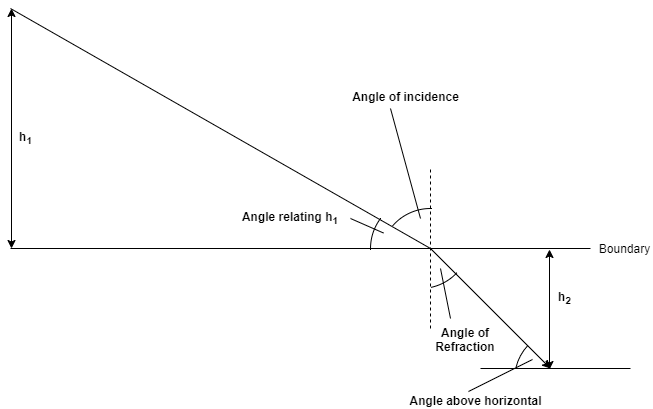# Problem: An underwater diver sees the sun 60° above horizontal.How high is the sun above the horizon to a fisherman in a boat above the diver? Express your answer using two significant figures.

###### FREE Expert SolutionThe angle above horizontal = 60°

We'll define the angle of incidence as the angle at which the ray from the sun strikes the interface.

The boat is at the boundary. Therefore, the target is angle relating h1.

The angle of refraction = 90 - 60 = 30°

Use Snell's Law to relate the Angle of Incidence with the Angle of Refraction:

99% (94 ratings)###### Problem Details

An underwater diver sees the sun 60° above horizontal.

How high is the sun above the horizon to a fisherman in a boat above the diver? Express your answer using two significant figures.